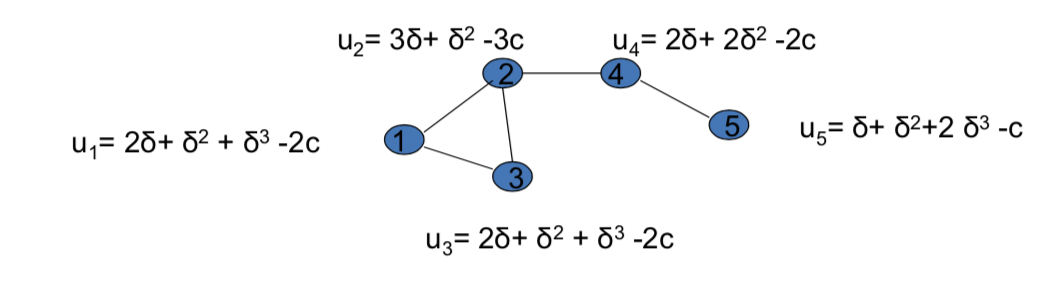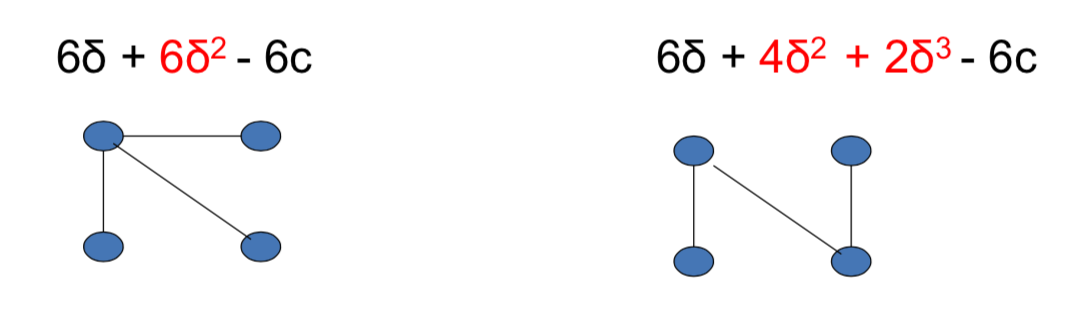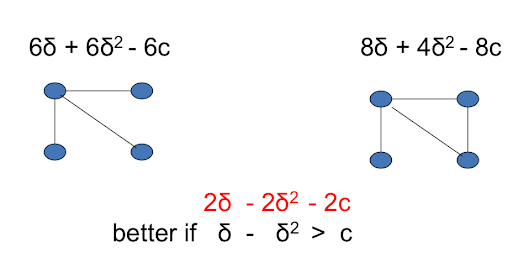## Connections Model JW96¶

• $0 \leq \delta_{ij} \leq 1$ a benefit parameter for i from connection between i and j
• $0 \leq c_{ij}$ cost to i of link to j
• $\iota(i, j)$ shortest path length between i, j
• $u_i(g) = \sum_j\delta_{ij}^{\iota(i, j)} - \sum_{j.in.N_i(g)}C{ij}$

## Symmetric Version:¶

• benefit from a friend is $\delta < 1$
• benefit from a friend of a friend is $\delta^2$,...
• cost of a link is $c>0$So, one nice thing about this type of approach is that once we've specified utilities and welfare calculations for each individual. Then, we end up having the ability to evaluate networks and say which ones are good and which ones are bad, directly by doing calculations in terms of the welfare properties. And this is something that's very different from the random network.

So, is a network formed by preferential attachment better than a network that is formulated at random? Well, we have no idea because we didn't have any specification of what that did for the individuals involved. A . We value indirect friendships at a certain level, and once we've done that, now we can assign values to these things and then make welfare evaluations. So, it's not only that by doing this we get predictions about which ones are going to form, but we also can evaluate them and so that's an important aspect of this.

And one other thing to say in terms of pair y stability and this idea of forming networks strategically. It's not necessarily true that each individual has to be Machiavellian or, calculating about all their friendships or I want to form this friendship because it's beneficial and that one because it's not.

It's more just that what's necessary is that individuals will tend to form things which are beneficial and when things aren't working out, they tend to get rid of them. So as long as their pressures in those directions. Then we can talk about dynamics, and so forth afterwards. But that'll give some push towards these kinds of networks, and these are ones that are going to be stable, in the sense that nobody then has an incentive to move away from these. So they'll be rest points of different processes, where people don't have to be so calculating, but at least pushed in the right directions, and eventually reach these kind of networks.

## Efficient Networks in the Symmetric Connections Model¶

• low cost: $c < \delta - \delta^2$
• complete network is uniquely efficient
• that's going to be the situation where it's more beneficial to have a direct relationship. This is the value for direct relationship($\delta$) compared to an indirect relationship of distance 2($\delta^2$). So shortening anything of distance 2 or even further, the gain in changing that is bigger thant he loss in terms of cost of adding a link. So adding a link is always going to be beneficial and the complete network is going to be uniquely efficient in that setting.
• medium cost: $\delta - \delta^2 < c < \delta + (n - 2)\delta^2/2$
• star networks with all agents are uniquely efficient
• So the only architecture which is going to be efficient is going to be have somebody in the center and then everybody else have that one link to that person, and that's going to be the thing that maximizes total sum of utilizes necessity, and the only thing which maximiazes the total sum of utilies.
• high cost: $\delta + (n - 2)\delta^2 / 2 < c$
• empty network is uniquely efficient
• it just makes sense that nobody should connect any links, just too expensive it doesn't make sense to have anybody talking to anybody. So for very high costs the empty network is uniquely efficient

So the meat of understanding this is really going to be understanding this middle part(medium cost) because the other extremes(low cost and high cost) are going to be fairly.

As long as you get a higher benefit from a direct connection, and a lower benefit from an indirect connection, and a lower benefit from things. There won't be anything special about it being $\delta$, $\delta^2$ and $\delta^3$, it just has to be some value, some value for two distance, a value for three distance, and you'll see that everyghing we say in all the proofs and so forth would go through if you just separated put in, subsituted something else for the $\delta$ and so forth

## Why Stars?¶

What's important is the indirect benefits that flow through the network generate extra value## Star versus Complete:¶So what we've done is we've moved some of the distance 2 things, over to direct connections, but we've paid a couple of extra costs of maintaining links to do so